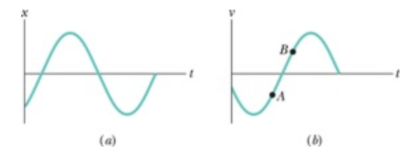# Problem: Which of the following describe φ for the SHM x(t) = acos(ωt + φ) of figure (a)?a. π/2 &lt; φ &lt; πb. π &lt; φ &lt; 3π/2c. - 3π/2 &lt; φ &lt; - π

###### FREE Expert Solution

The solution of the motion is x(t) = acos(ωt + φ)

The amplitude of the wave is

At t = 0, the value of x(t) is 0 < x(t) < -a. That is, the value of x(t) is between 0 and -a.

99% (284 ratings)###### Problem DetailsWhich of the following describe φ for the SHM x(t) = acos(ωt + φ) of figure (a)?

a. π/2 < φ < π

b. π < φ < 3π/2

c. - 3π/2 < φ < - π